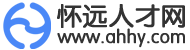• 微信
• 登录 | 注册• 不限
• 市场销售
• 行政人事
• 城建装修
• 客户服务
• 会计财务
• 普工技工
• 教育培训
• 质控安防
• 设计包装
• 百货零售
• 贸易采购
• 餐饮休闲
• 金融保险
• 电子电气
• 房产中介
• 司机后勤
• 物流贸易
• 农林牧渔
• 物业管理
• 医院护理
• 保健按摩
• 运动健身
• 环境环保
• 美容美发
• 酒店旅游
• 法律产权
• 外语翻译
• 娱乐休闲
• 汽车服务
• 高级管理
• 淘宝职位
• 生产管理
• 服装纺织
• 生物制药
• 市场媒介
• 广告会展
• 机械仪器
• 网络通信
• 家政安保
• 编辑发行
• 其他分类

• 不限
• 英语翻译
• 日语翻译
• 韩语翻译
• 法语翻译
• 俄语翻译
• 德语翻译
• 西班牙语翻译
• 意大利语翻译
• 葡萄牙语翻译
• 阿拉伯语翻译
• 小语种翻译

• 不限
• 怀远县城
• 蚌山区
• 淮上区
• 禹会区
• 龙子湖区
• 龙亢镇
• 包集镇
• 河溜镇
• 白莲坡镇
• 常坟镇
• 唐集镇
• 双桥集镇
• 魏庄镇
• 万福镇
• 褚集镇
• 淝南镇
• 古城镇
• 陈集镇
• 淝河乡
• 徐圩乡
• 兰桥乡
• 其他地区

• 不限
• 今天
• 三天内
• 一周内
• 一个月内
• 三个月内

• 不限
• 全职
• 兼职

• 不限
• 1000元以下
• 1000-2000元
• 2000-3000元
• 3000-5000元
• 5000-8000元
• 8000-1.2万元
• 1.2万-2万元
• 2万元以上
• 面议抱歉！在怀远人才网里没有找到与筛选相关的职位